Scenario:

The dataset has sales transactions data with transaction date.

Requirement:

Derive the week start date and week end date for a given transaction date considering the week starts from ‘Sunday’.

Deriving Start Date of a Week:

1.   To derive the start date, the first step is to extract the day of the week from the date column (in the dataset), as the rest of the calculations are dependent on this day number.

In Smarten, output for Day of Week is based on following order:

 1 = Sunday 5 = Thursday 2 = Monday 6 = Friday 3 = Tuesday 7 = Saturday 4 = Wednesday

Steps:

Right click on Date column -> Add column -> Day of Week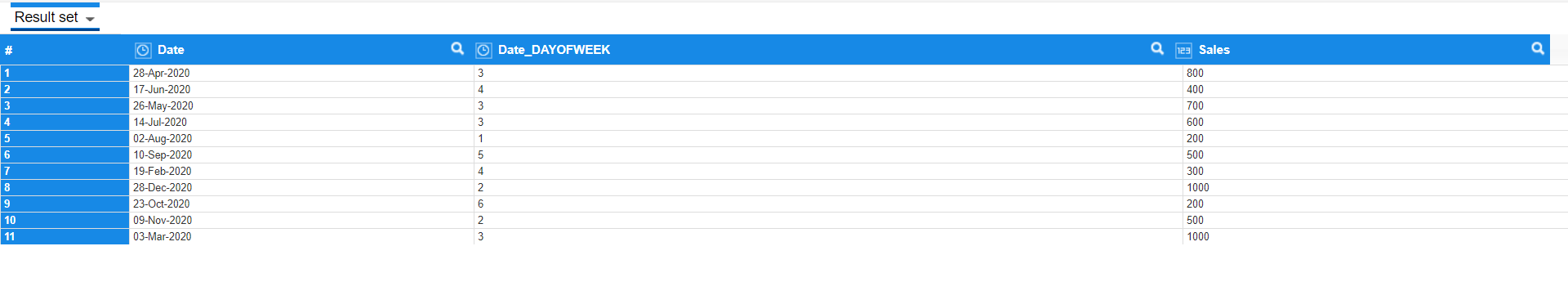For example:

 Date Day Of Week 28-Apr-2020 3 (Tuesday) 17-Jun-2020 4 (Wednesday) 02-Aug-2020 1 (Sunday)

2.   To get the week start day, we need to reach up to the date that falls under Sunday of the same week. To achieve the same, we need to subtract the ‘week day number -1’ from the current date.

Week day number – 1 calculation will derive a new week day number as given in the below example.

Example:

 Date Current Day Of Week New Day Of Week 28-Apr-2020 3 (Tuesday) 2 (Monday) 17-Jun-2020 4 (Wednesday) 3 (Tuesday) 02-Aug-2020 1 (Sunday) 0

In the above example, the day of week of 28th April 2020 is 3 as it falls under Tuesday.

After Subtracting 1 from the Day of Week, it would be 2.

Steps:

By using Transform -> Minus () function we cansubtract 1 from weekday number.

Right click on the Day of week column-> Transform -> More ->minus (number, number)

In the transform operation, select Argument 1 as‘Day of Week’ column and Argument 2 as 1 in the static value and APPLY.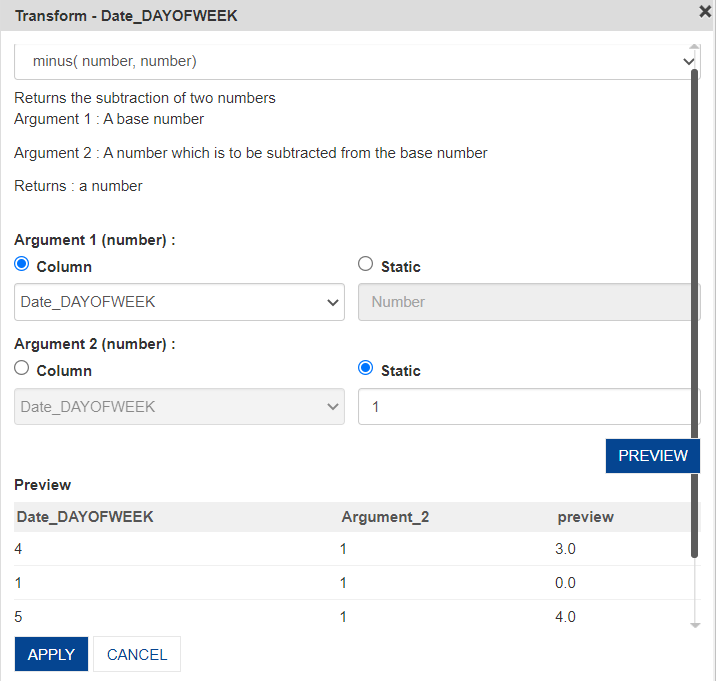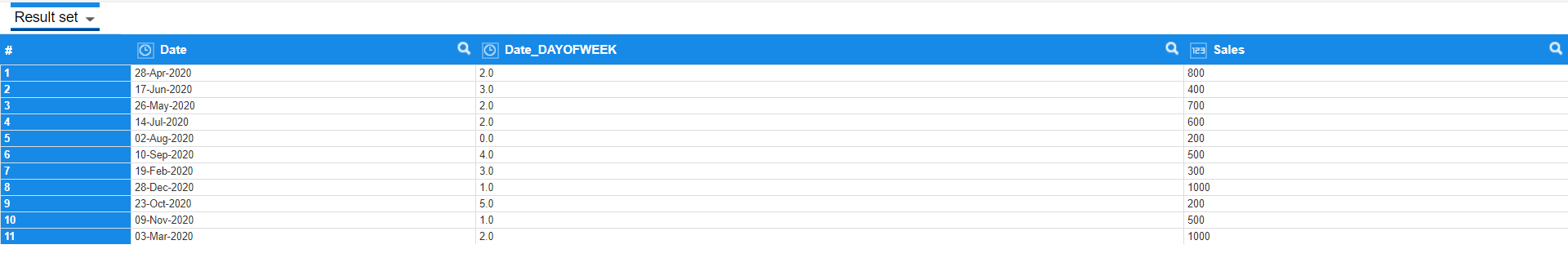3.   As a next step we need to subtract the New week number (that was derived in step 2) from the transaction date to get the week start day.

When using dateAdd() function to calculate a previous date, the number has to be taken as a negative number (Here subtraction operation will be performed though we are using DateAdd()).

To do the same, you need to multiply the week day number by -1.

Steps:

Right click on Day of week column-> Transform ->More->multiply (number, number)

In the Transform for Multiply window, give Argument 1 as Day of Week and Argument 2 as-1 in the static value section (as we are multiplying each week day by-1).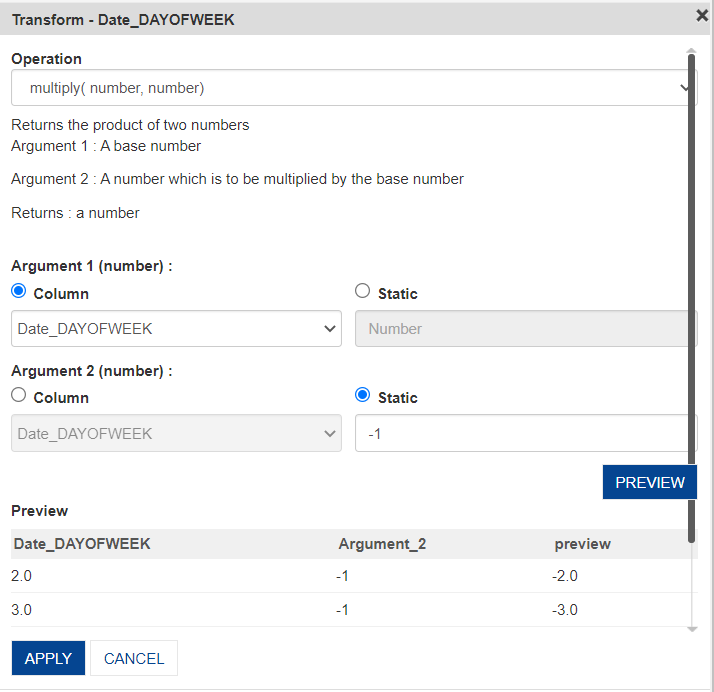Example:

 Date Current Day Of Week NewDay Of Week 28-Apr-2020 2 -2 17-Jun-2020 3 -3 02-Aug-2020 0 0

4.   Make a copy of the current Date column to calculate the week start date in a new column.

Right click -> Copy ->Column (Date column)

The new date column will be created as below (Date_1).5.   Now, to get the week start date that falls under Sunday, the number of days has to be added (which is the new week day number derived in Step 3) from the date column.

Example: Consider the transaction date as 28th Apr 2020 which falls under Tuesday. The newly derived week day (ie; -2) has to be added to get the date that falls on Sunday which would be 26th April 2020.

Steps:

Right click on the copied Date column-> Transform -> more ->dateAdd()

In the transform window, give Argument 1as Day, Argument 2 as Day of Week column (that is our no. of days to be subtracted)andArgument 3 as the new copied date column (where we want the output).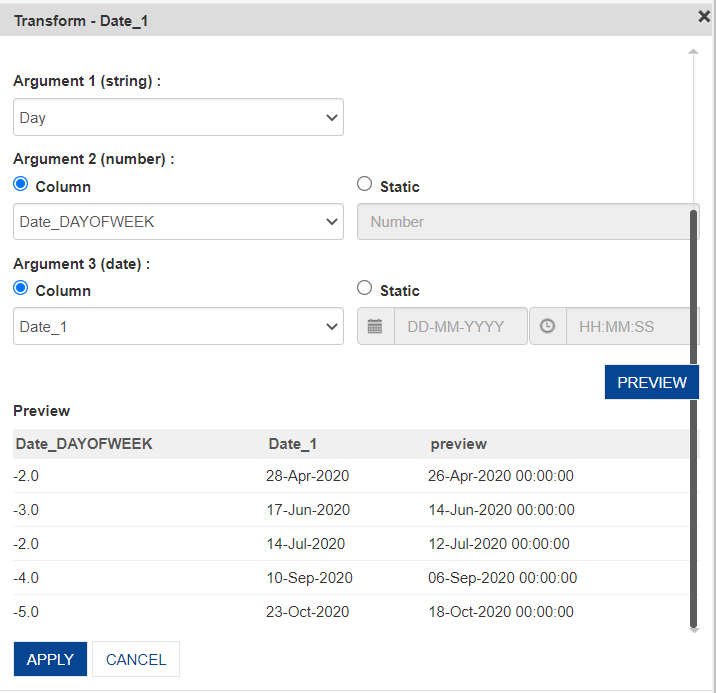Week start date will be generated as below.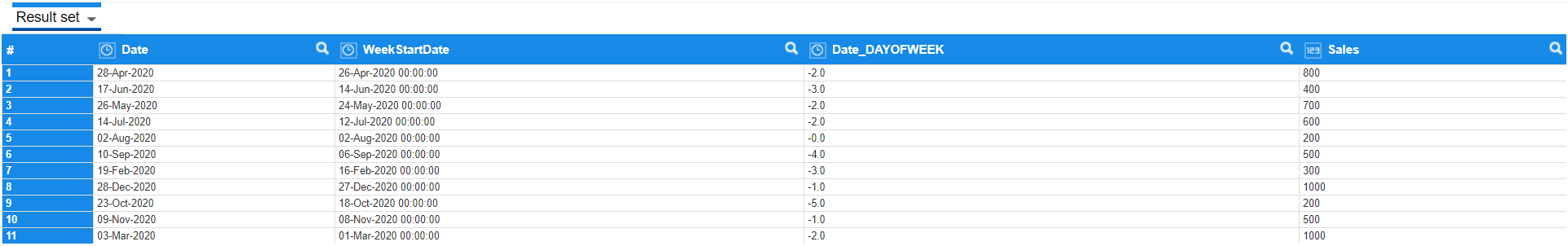Example:

 Date Day Of Week Start Date Column 28-Apr-2020 -2 26-Apr-2020 17-Jun-2020 -3 14-Jun-2020 02-Aug-2020 0 02-Aug-2020

Deriving week end date:-

Once the week start date is ready, it is easy to derive the week end date as it falls after 6 days of the start date.

1.   Take a copy of the week start date column.

Right click (week start date column) -> copy -> column.

2.   Now, we just have to add 6 days to the start date to get the end date of the week.

Example: If the start date is 26th Apr 2020, after adding 6 days, the week end date (2nd May 2020) will be derived.

Steps:

Right click on the week start date column -> Transform -> more ->dateAdd.

In the Transform window, give Argument 1 as Day, Argument 2as 6 in the static option (as we are adding 6 days to get week end dates)and Argument3 as the new copied date column.The week end date column will be derived as below.Example:

 Date Start Date Column End Date Column 28-Apr-2020 26-Apr-2020 02-May-2020 17-Jun-2020 14-Jun-2020 20-Jun-2020 02-Aug-2020 02-Aug-2020 08-Aug-2020

This way, we can derive the start date and end date of the week from any random week date.